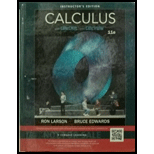Chapter 11.5, Problem 56E### Calculus (MindTap Course List)

11th Edition
Ron Larson + 1 other
ISBN: 9781337275347

#### Solutions

Chapter
Section### Calculus (MindTap Course List)

11th Edition
Ron Larson + 1 other
ISBN: 9781337275347
Textbook Problem

# Finding an Equation of a PlaneIn Exercises 45–56, find an equation of the plane with the given characteristics.The plane passes through the points ( 4 , 2 , 1 ) and ( − 3 , 5 , 7 ) and is parallel to the z-axis.

To determine

To calculate: The equation of the plane passing through the points (4,2,1) and (3,5,7).

Explanation

Given:

The plane passes through the point (4,2,1) and (3,5,7), and is parallel to the z-axis.

Formula used:

The standard equation of the plane is given by:

a(xx1)+b(yy1)+c(zz1)=0

Calculation:

To determine equation of a plane the point through which it is passing and the normal vector to the plane should be known.

Two choices for the point but there is no normal vector provided.

Since, the required plane is parallel to z-axis, so the normal vector will be obtained by the cross product of u 0,0,1 and the vector v which is extending from the point (4,2,1) to the point (3,5,7).

So, component form of v will be

v=34,52,71=7,3,6

So, it follows that a vector normal to the required plane is

n=u×v=|ijk0017

### Still sussing out bartleby?

Check out a sample textbook solution.

See a sample solution

#### The Solution to Your Study Problems

Bartleby provides explanations to thousands of textbook problems written by our experts, many with advanced degrees!

Get Started

## Additional Math Solutions

#### Find more solutions based on key concepts